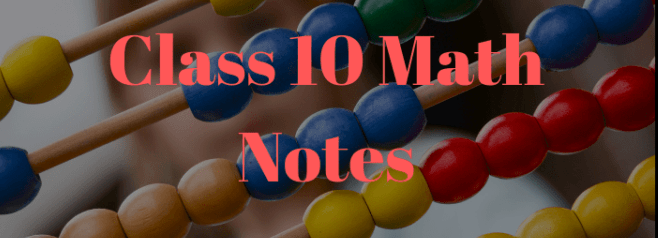## Class 10 Maths Coordinate Geometry Handwritten Notes by Toppers – Download PDF

In the field of mathematics, coordinate geometry plays a crucial role in understanding the relationship between algebraic equations and geometric shapes. For students studying in Class 10, having comprehensive and reliable study materials is essential for grasping the concepts effectively. One valuable resource that can greatly aid in learning is the handwritten notes prepared by the top-performing students. In this article, we will explore the significance of Class 10 Maths Coordinate Geometry handwritten notes, their benefits, and provide a convenient way to access and download them.

Class 10 Maths Coordinate Geometry – Get here the Handwritten Notes for Class 10 Coordinate Geometry. Candidates who are ambitious to qualify the Class 10 with a good score can check this article for Notes. Below we provided the link to access the Notes of Class 10 Maths for the topic Coordinate Geometry. You can practice the questions and check your answers from the solutions given after the question. By practicing these resources candidates definitely get the idea of which his/her weak areas and how to prepare well for the examination.

• Class: 10th
• Subject: Math
• Topic: Coordinate Geometry
• Resource: Handwritten Notes

Maths Handwritten Notes is based on the new(reduced) syllabus by CBSE.

# CBSE Class 10 Maths Coordinate Geometry Handwritten Notes

Particularly when it comes to the subject of Mathematics, students desire to have an answer key to help them in evaluating their learning and development. Refer to these solutions when practicing and solving the Mathematics exercises from NCERT Textbooks.

## Importance of Class 10 Maths Coordinate Geometry

Coordinate Geometry is a vital branch of mathematics that combines algebraic equations and geometric shapes. It provides a precise framework for analyzing and solving problems related to lines, angles, triangles, and more. Class 10 is a critical stage where students lay the foundation for more advanced mathematical concepts. Understanding coordinate geometry at this level is crucial as it forms the basis for higher-level studies in mathematics and other disciplines.

Handwritten notes have been proven to be an effective learning tool for students of all levels. Here are some advantages of using handwritten notes for studying:

1. Personalized Learning: Handwritten notes are prepared by students themselves, allowing them to tailor the content to their own understanding and learning style.
2. Active Engagement: Writing down notes encourages active engagement with the material, enhancing comprehension and retention.
3. Organization and Structure: Handwritten notes provide a well-organized structure, making it easier to review and revise the subject matter.
4. Focus and Attention: Taking notes requires concentration, leading to improved focus and enhanced learning outcomes.
5. Memorization: The process of writing helps reinforce memory, enabling students to recall information more effectively during exams.

## Benefits of Toppers’ Handwritten Notes

Toppers’ handwritten notes hold immense value for Class 10 students. Here are some benefits of utilizing these notes for studying coordinate geometry:

### Comprehensive Coverage of Topics

Toppers’ handwritten notes cover all the important topics and subtopics of coordinate geometry as per the Class 10 curriculum. These notes serve as a reliable resource for understanding concepts thoroughly.

### Clear and Concise Explanation

The notes provided by top-performing students offer clear and concise explanations of various concepts. They simplify complex ideas and present them in a manner that is easy to understand, facilitating effective learning.

### Step-by-Step Problem Solving

Coordinate geometry often involves solving problems and equations. Toppers’ handwritten notes provide step-by-step solutions to example problems, enabling students to grasp the problem-solving techniques required in this field.

### Visual Representation of Concepts

Visual aids play a vital role in understanding geometric concepts. Toppers’ handwritten notes include diagrams, graphs, and illustrations, helping students visualize and comprehend geometric shapes and their relationships with algebraic equations.

## Conclusion

Class 10 Maths Coordinate Geometry handwritten notes by toppers offer an excellent resource for students aiming to excel in their studies. The personalized touch and expertise of top-performing students make these notes highly valuable in understanding the fundamental concepts of coordinate geometry. By accessing and utilizing these handwritten notes, students can enhance their understanding, improve problem-solving skills, and build a solid foundation for further studies in mathematics.